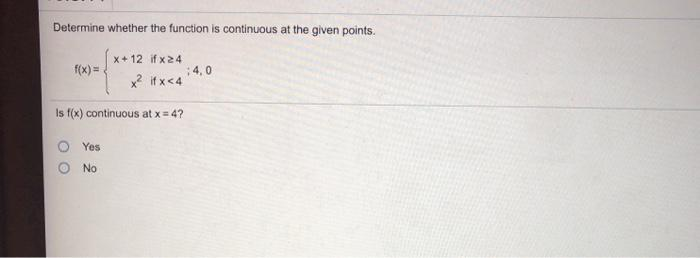### Create an Account

Home / Questions / Determine whether the function is continuous at the given points. f(x)= X+ 12 if x 24 x² i...

# Determine whether the function is continuous at the given points. f(x)= X+ 12 if x 24 x² if x 54 4,0 Is f(x) continuous at x = 4? Yes No

Determine whether the function is continuous at the given points. f(x)= X+ 12 if x 24 x² if x 54 4,0 Is f(x) continuous at x = 4? Yes NoApr 19 2021 View more View LessSubscribe To Get Solution